# Partitioning Numbers into Hundreds, Tens and Units

Partitioning Numbers into Hundreds, Tens and Units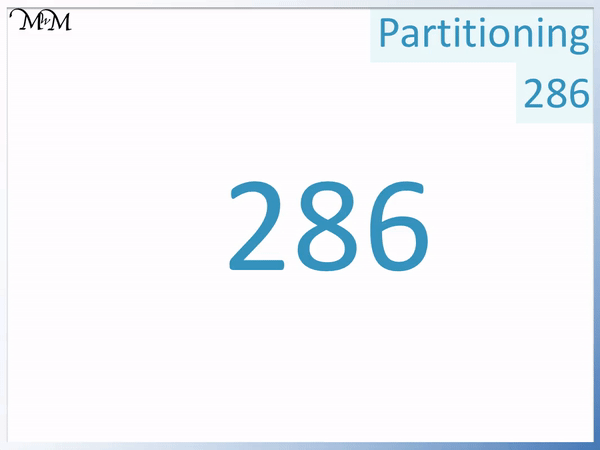• Partitioning a number is splitting it up into the sum of smaller numbers.
• Partitioning a number into its hundreds, tens and units will separate the number into the values represented by each digit.
• The ‘2’ in 285 represents 200.
• The ‘8’ in 285 represents 80.
• The ‘5’ is in the ones column and is just worth 5.
• We write the larger number in expanded form as a sum of the partitioned values.
• 285 = 200 + 80 + 5.
• Writing a number in expanded form means to write the number as the sum of the values of each digit.

We write the number as the sum of the values represented by each digit.• We will partition the number 574 into its hundreds, tens and units and write it in expanded form.
• The ‘4’ in in the units (or ones) column and is just worth 4.
• The ‘7’ is in the tens column and so, 7 tens are worth 70.
• The ‘5’ is in the hundreds column and 5 hundreds are worth 500.
• 574 is written in expanded form as 500 + 70 + 4.
• We have partitioned the larger number into the sum of its hundreds, tens and ones.Supporting Lessons#### Partitioning Numbers Interactive Arrow Cards

Partitioning Arrow Cards (2-Digit Numbers): Interactive Questions

# Partitioning Numbers into Hundreds, Tens and Units and Writing them in Expanded Form

Partitioning a number means to write it as the sum of smaller numbers.

Partitioning is an important method because breaking down larger numbers into smaller numbers means that they are easier to work with when performing calculations.

To partition a number into its hundreds, tens and units, we write the number as the sum of the values of its digits.

Writing a number in expanded form means to write the number as the sum of the values of its digits.

We will start by looking at an example of partitioning a number with only 2 digits.

The number 12 has 2 digits which are ‘1’ in the tens column and ‘2’ in the units column.

The ‘1’ ten is worth 10 and the ‘2’ units are just worth 2.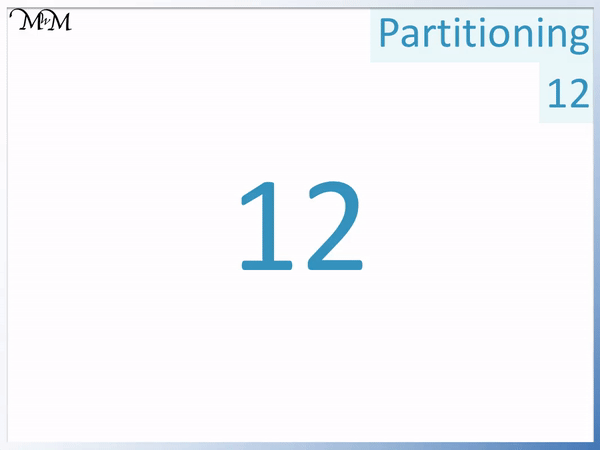12 can be partitioned into its tens and units as 10 + 2.

10 is the value of the digit ‘1’ and 2 is the value of the digit ‘2’. Writing a number as the sum of the value of its digits is called writing the number in expanded form.We will now partition the number of 25 into its tens and units, writing it in expanded form.

The digit of ‘5’ is in the units column as it is the rightmost digit. It is just worth 5.

The digit of ‘2’ is in the tens column. 2 tens are worth 20.

We can also see that the ‘2’ in 25 is worth 20 by writing 25 with a zero in the place of the 5.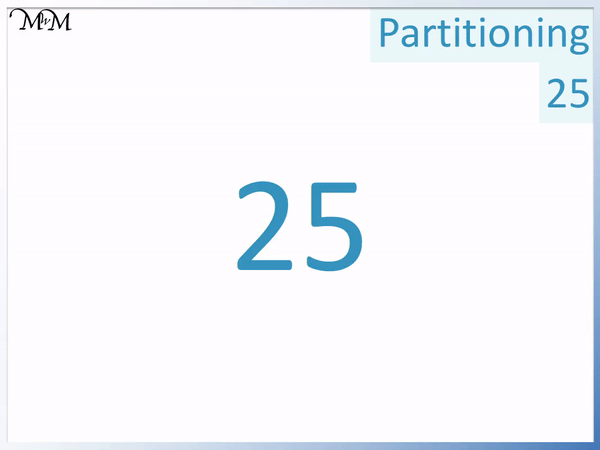We can write 25 in expanded form as 20 + 5.

We can write 25 = 20 + 5.Here is another example of partitioning into tens and ones.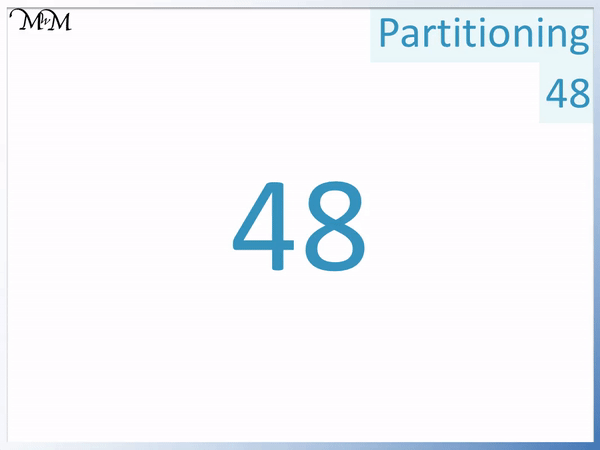48 contains two digits, which are a ‘4’ in the tens column and an ‘8’ in the ones column.

4 tens are worth 40 and 8 ones are worth 8.

48 = 40 + 8

We have partitioned 48 its expanded form notation.We will now partition 93 into its tens and units.9 tens are worth 90 and 3 units are worth 3.

We can partition 93 into its tens and units and write it in expanded form as:

93 = 90 + 3We have looked at partitioning two-digit numbers into tens and units.

We will now look at partitioning three-digit numbers into their hundreds, tens and units.

The first example of partitioning a 3-digit number will be to partition the number 123.

The ‘1’ in 123 is in the hundreds column and 1 hundred is worth 100.

The ‘2’ in the tens column is worth 2 tens, which is 20.

The’3′ is in the ones column and so it is just worth 3.We write the number in expanded form as the sum of the values of these digits above.

123 = 100 + 20 + 3The digit of ‘1’ in 123 is worth 100 and this can be seen by replacing the other digits after the ‘1’ in 123 with zeros.

The digit of ‘2’ is worth 20 and this can be seen by replacing the digit after ‘2’ in 123 with a zero.

Here is another example of writing a three-digit number in expanded form using the partitioning method.

We will partition 286 into hundreds, tens and units.The digit of ‘2’ in 286 is in the hundreds column.

2 hundreds = 200.

The digit of ‘8’ in 286 is in the tens column.

8 tens = 80.

The digit of ‘6’ in 286 is in the units column.

6 units = 6.We will partition the 3-digit number of 574 into expanded form as the sum of its hundreds, tens and units.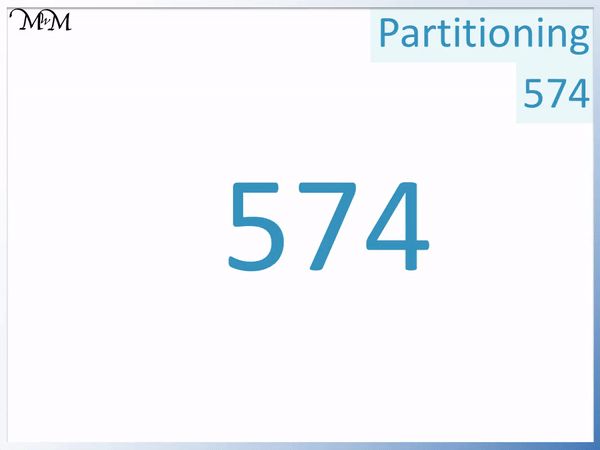The digit of ‘5’ is worth 500.

The digit of ‘7’ is worth 70.

The digit of ‘4’ is just worth 4.In expanded form, 574 = 500 + 70 + 4.

It has been partitioned into the hundreds, tens and units place value columns.

In this next example we look at the number 305.

305 is a three-digit number which has the three digits of ‘3’, ‘0’ and ‘5’.

The ‘3’ is in the hundreds column and is worth 300.

The ‘0’ is in the tens column and is worth 0.

The ‘5’ is in the ones column and so is worth 5.

In this example we have a ‘0’ in the tens and because this digit holds no value, we do not write it.We partition 305 into the sum of 300 and 5.

We do not write the tens column in this sum because it is worth zero. We simply skip it.

In expanded form we can write:

305 = 300 + 5

We do not write 300 + 0 + 5 as there is no need to write the tens place value column in this example.

We never include digits with a ‘0’ in our expanded form answer.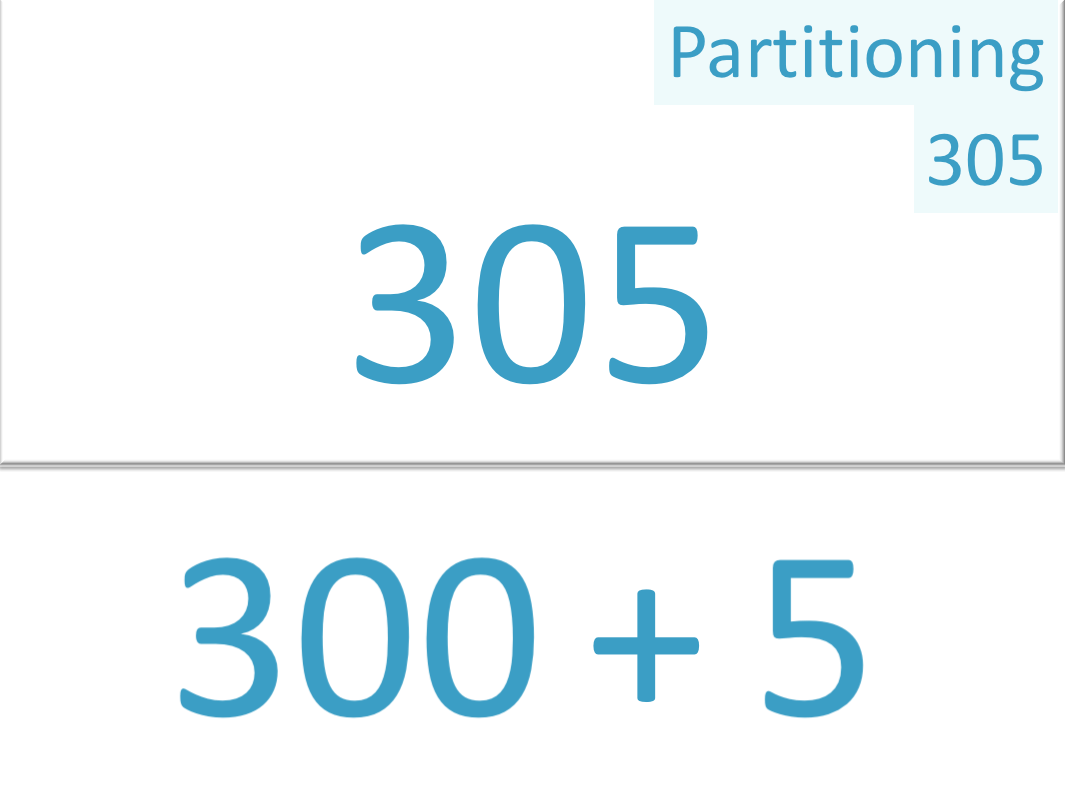When teaching partitioning it can be useful to use partitioning arrow cards to help visualise the value of each digit.Now try our lesson on Multiplication by Partitioning where we learn how to use partitioning to multiply larger numbers.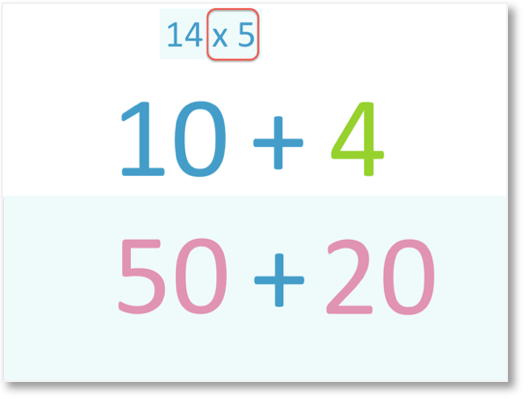error: Content is protected !!